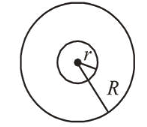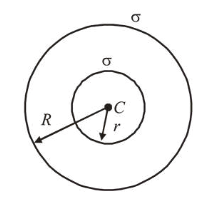# A charge Q is distributed over two concentric conducting`
Question:

A charge $Q$ is distributed over two concentric conducting thin spherical shells radii $r$ and $R(R>r)$. If the surface charge densities on the two shells are equal, the electric potential at the common centre is :1. (1) $\frac{1}{4 \pi \varepsilon_{0}} \frac{(R+r)}{2\left(R^{2}+r^{2}\right)} Q$

2. (2) $\frac{1}{4 \pi \varepsilon_{0}} \frac{(2 R+r)}{\left(R^{2}+r^{2}\right)} Q$

3. (3) $\frac{1}{4 \pi \varepsilon_{0}} \frac{(R+2 r) Q}{2\left(R^{2}+r^{2}\right)}$

4. (4) $\frac{1}{4 \pi \varepsilon_{0}} \frac{(R+r)}{\left(R^{2}+r^{2}\right)} Q$

Correct Option: , 4

Solution:

(4) Let $\sigma$ be the surface charge density of the shells.Charge on the inner shell, $Q_{1}=\sigma 4 \pi r^{2}$

Charge on the outer shell, $Q_{2}=\sigma 4 \pi R^{2}$

$\therefore$ Total charge, $Q=\sigma 4 \pi\left(r^{2}+R^{2}\right)$

$\Rightarrow \sigma=\frac{Q}{4 \pi\left(r^{2}+R^{2}\right)}$

Potential at the common centre,

$V_{C}=\frac{K Q_{1}}{r}+\frac{K Q_{2}}{R}$             $\left(\right.$ where $\left.K=\frac{1}{4 \pi \varepsilon_{0}}\right)$

$=\frac{K \sigma 4 \pi r^{2}}{r}+\frac{K \sigma 4 \pi R^{2}}{R}=K \sigma 4 \pi(r+R)$

$=\frac{K Q 4 \pi(r+R)}{4 \pi\left(r^{2}+R^{2}\right)}=\frac{1}{4 \pi \varepsilon_{0}} \frac{(r+R) Q}{\left(r^{2}+R^{2}\right)}$